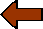Clinical Laboratory - FundamentalsReturn to the main menu

Sensitivity, Specificity, and Predictive Values

Sensitivity and Specficity

For the interpretation of test findings:

Sensitivity= true positives / (true positives + false negatives)

Specificity = true negatives / (true negatives + false positives)

Sensitivity is the ability to detect a disease if it is really present. Can the test find the abnormality?

Specificity is the ability to exclude persons who do not have the disease. Can the test results exclude those persons without the disease?

Therefore, the best screening test must have high sensitivity, to detect as many people as possible with the disease. However, this initial screening test is followed by a confirmatory test with high specificity to ensure that a positive test detected with screening is a true positive.

Negative and Positive Predictive Value

Positive Predictive Value = True positives / True positives + False positives

The positive predictive value tells you how often a positive test represents a true positive.

How likely is a positive test to indicate that the person has the disease?

Negative Predictive Value = True negatives / True negatives + False negatives

The negative predictive value tells you how often a negative test represents a true negative.

How likely is a negative test to indicate that the disease is not present in the person?

Predictive values are based upon the prevalence of disease in a population. As the prevalence of disease decreases, the positive predictive value decreases. In the general population, few diseases reach a prevalence of 1%.

For example, if a test has 95% sensitivity and 95% specificity (considered very good), then:

Predictive values are based upon the prevalence of disease in a population. For a test with 99% sensitivity and 99% specificity, here are positive predictive values for different levels of prevalence.

 Prevalence of Disease (%) Positive Predictive Value (%) 1 16 2 28 5 50 10 68 25 86 50 95

Therefore, at low disease prevalence, the negative predictive value of a test is always higher than the positive predictive value. It is always easier to exclude a disease with a negative test. Therefore, a negative test result has value to exclude a disease. Most positive results, however, will be false positives, so more information is needed for a positive test, such as confirmatory testing, either repeating the test or performing a test with greater specificity.

You can improve predictive value by first narrowing down the population to be tested with standard history and physical exam (e.g., don't order superfluous lab tests).

Example: you can progressively improve you chances of getting a meaningful result for a prostate specific antigen test if you order it on: men (this should be obvious), older men, older men with a palpable nodule.

 Which of the following is most important for increasing predictive value of a positive test result? A Disease prevalence B Disease severity C Test sensitivity D Test specificity